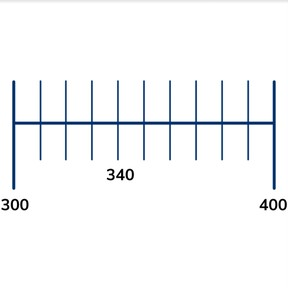Neighboring hundreds numbers

# Neighboring hundreds numbers

Neighboring hundreds numbers

No account needed.8,000 schools use Gynzy92,000 teachers use Gynzy1,600,000 students use Gynzy

## General

Students learn what neighboring hundreds numbers are and can determine the neighboring hundreds numbers of numbers from 101 to 999 by determing the hundreds number before and after the given number.

2.NBT.A.2

## Relevance

Discuss with students that it is important to know neighboring hundreds numbers because you then know where a number fits. Explain that you can use this to find a car in a parking garage.

## Introduction

Students must answer true or false to a number of statements. Drag the cover to show the next statement.

## Development

Explain what hundreds are. A hundreds is a group of 100 and always ends on 00. Name the hundreds numbers. Next explain that neighboring hundreds numbers are the hundreds numbers that a given number sits between. Using the number line, show that 340 sits between 300 and 400. Next explain that you can find neighboring hundreds numbers by placing the number on the number line and looking at which hundreds number comes before and which comes after the given number. Next ask students what the neighboring hundreds numbers are for the given numbers and erase the boxes to show the answers.

Check that students understand neighboring hundreds numbers by asking the following questions:
- What are neighboring hundreds numbers?
- How do you find neighboring hundreds numbers?
- Do all numbers have neighboring hundreds numbers?

## Guided Practice

Students first practice finding neighboring hundreds numbers from multiple given answers. Then they must determine neighboring hundreds numbers on their own.

## Closing

Check that students can explain the idea of neighboring hundreds numbers. Tell students that the neighboring hundreds numbers are the hundreds numbers that a given number sits between. Emphasize that it is useful to know neighboring hundreds numbers to better know where a number sits. This is useful in a parking garage. Finally, check that students can find the neighboring hundreds numbers of given numbers. Give students cards with the hundreds on them in groups. Give the students a number and ask them to find the neighboring hundreds numbers in groups. Have them hold up their answers.

## Teaching Tip

For students who have difficulty finding the neighboring hundreds numbers, have them use a number line to help visualize the process.

## Instruction materials

Number cards showing the hundreds for each group.

### The online teaching platform for interactive whiteboards and displays in schools

• Save time building lessons

• Manage the classroom more efficiently

• Increase student engagement